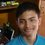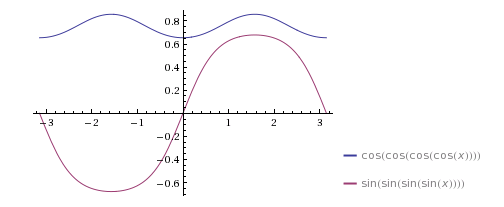# Trig in trig in trig in trig

Can anyone please explain how I can solve the following problem:

Solve for $x$:

$\cos(\cos(\cos(\cos x))) = \sin(\sin(\sin(\sin x)))$Note by Nilabha Saha
4 years, 8 months ago

This discussion board is a place to discuss our Daily Challenges and the math and science related to those challenges. Explanations are more than just a solution — they should explain the steps and thinking strategies that you used to obtain the solution. Comments should further the discussion of math and science.

When posting on Brilliant:

• Use the emojis to react to an explanation, whether you're congratulating a job well done , or just really confused .
• Ask specific questions about the challenge or the steps in somebody's explanation. Well-posed questions can add a lot to the discussion, but posting "I don't understand!" doesn't help anyone.
• Try to contribute something new to the discussion, whether it is an extension, generalization or other idea related to the challenge.
• Stay on topic — we're all here to learn more about math and science, not to hear about your favorite get-rich-quick scheme or current world events.

MarkdownAppears as
*italics* or _italics_ italics
**bold** or __bold__ bold
- bulleted- list
• bulleted
• list
1. numbered2. list
1. numbered
2. list
Note: you must add a full line of space before and after lists for them to show up correctly
paragraph 1paragraph 2

paragraph 1

paragraph 2

[example link](https://brilliant.org)example link
> This is a quote
This is a quote
    # I indented these lines
# 4 spaces, and now they show
# up as a code block.

print "hello world"
# I indented these lines
# 4 spaces, and now they show
# up as a code block.

print "hello world"
MathAppears as
Remember to wrap math in $$ ... $$ or $ ... $ to ensure proper formatting.
2 \times 3 $2 \times 3$
2^{34} $2^{34}$
a_{i-1} $a_{i-1}$
\frac{2}{3} $\frac{2}{3}$
\sqrt{2} $\sqrt{2}$
\sum_{i=1}^3 $\sum_{i=1}^3$
\sin \theta $\sin \theta$
\boxed{123} $\boxed{123}$

## Comments

Sort by:

Top Newest

What have you done? What have you tried? Where are you stuck?

Staff - 4 years, 8 months ago

Log in to reply

This is a problem which apparently came in one of Russia's elite math examinations (I have no idea which one) Source: The Art And Craft Of Problem Solving

- 4 years, 8 months ago

Log in to reply

However, I graphed these two functions and found that they never intersect, implying there are no solutions. But, how do we theoretically prove that this has no solutions within the real numbers?

- 4 years, 8 months ago

Log in to reply

Yup, the idea is to show that these 2 graphs never intersect (over the reals). It's somewhat tricky to prove that though. AFAIK there isn't a "non-calculus" approach.

Staff - 4 years, 8 months ago

Log in to reply

Can you guide me on the calculus approach?

- 4 years, 8 months ago

Log in to reply

Well, according to Wolfram alpha, the solution(s) are:

\begin{aligned} x & \approx & -4.71 \pm 1.21 i \\ x & \approx & 0.76 \pm 0.61i \end{aligned}

Its graph looks like this:I couldn't properly solve the problem, but I managed to get to this point.

$\cos(\cos(\cos(\cos x))) = \sin(\sin(\sin(\sin x))) \\ \implies \sqrt{1- \sin^2 \sqrt{1- \sin^2 \sqrt {1- \sin^2 \sqrt{1- \sin^2 x}}}} = \sqrt{1- \cos^2 \sqrt{1- \cos^2 \sqrt {1- \cos^2 \sqrt{1- \cos^2 x}}}} \\ \implies \sin^2 \sqrt{1- \sin^2 \sqrt {1- \sin^2 \sqrt{1- \sin^2 x}}} = \cos^2 \sqrt{1- \cos^2 \sqrt {1- \cos^2 \sqrt{1- \cos^2 x}}} \\ \implies \cos^2 \sqrt{1- \cos^2 \sqrt {1- \cos^2 \sqrt{1- \cos^2 x}}} - \sin^2 \sqrt{1- \sin^2 \sqrt {1- \sin^2 \sqrt{1- \sin^2 x}}} = 0 \\ \implies \bigg[ \cos \left( \sqrt{1- \cos^2 \sqrt {1- \cos^2 \sqrt{1- \cos^2 x}}} + \sqrt{1- \sin^2 \sqrt {1- \sin^2 \sqrt{1- \sin^2 x}}} \right) \bigg] \cdot \bigg[ \cos \left( \sqrt{1- \cos^2 \sqrt {1- \cos^2 \sqrt{1- \cos^2 x}}} - \sqrt{1- \sin^2 \sqrt {1- \sin^2 \sqrt{1- \sin^2 x}}} \right) \bigg] = 0 \qquad \qquad \small \color{#3D99F6}{\text{Using} \ \cos^2 A - \sin^2 B = \cos(A+B) \cdot \cos(A-B)}$

Therefore, the trivial solutions are of form:

$1. \ \sqrt{1- \cos^2 \sqrt {1- \cos^2 \sqrt{1- \cos^2 x}}} + \sqrt{1- \sin^2 \sqrt {1- \sin^2 \sqrt{1- \sin^2 x}}} = \dfrac{(2n+1) \pi}{2} \qquad \small \color{#3D99F6}{\text{Where} \ n \in \mathbb{N}}$

$2. \ \sqrt{1- \cos^2 \sqrt {1- \cos^2 \sqrt{1- \cos^2 x}}} - \sqrt{1- \sin^2 \sqrt {1- \sin^2 \sqrt{1- \sin^2 x}}} = \dfrac{(2m+1) \pi}{2} \qquad \small \color{#3D99F6}{\text{Where} \ m \in \mathbb{N}}$

The two pairs of answers which are complex will be of the forms as discussed above.

I don't know of a better way to approach this problem.

- 4 years, 8 months ago

Log in to reply

Is there any other way? Perhaps, since we know that sin(x) = cos(x) for x = \frac{{pi}{4} and \frac{5 * {pi}}{4}. Or that sin({pi} - x) = cos(x). Do we get any lead like that? I couldn't find any, but you might. Thanks for helping.

- 4 years, 8 months ago

Log in to reply

Well, you might get principal solutions like that. But you have to think for general cases while solving problems like these, for example $\sin x = \cos x$ has the general solution of $n \pi + \dfrac{\pi}{4}$, where $n \in \mathbb{N}$. As for the identity $\sin^k \left( \dfrac{\pi}{2} - x \right) = \cos^k x$, I haven't still tried that approach. I would check it out too, and let you know.

I found it online that this is a problem from the All Russian Olympiad 1995, Grade 10, Problem 1.

- 4 years, 8 months ago

Log in to reply

Yes, I found the with the citation of 'Russia' in the book 'The Art And Craft Of Problem Solving'.

- 4 years, 8 months ago

Log in to reply

×

Problem Loading...

Note Loading...

Set Loading...